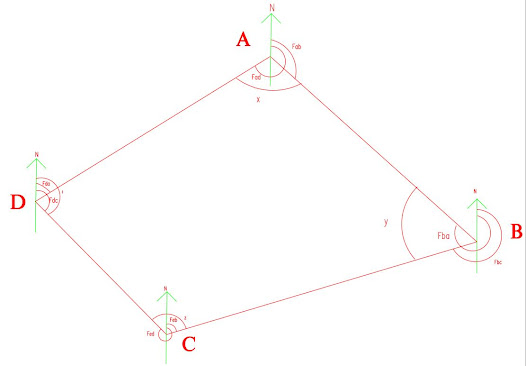# Methods of Traverse Surveying

Traverse surveying is a control survey which involves a number of survey lines forming a framework . The survey lines and directions are measured using distance measuring devices and angle measuring devices.

Usually, it is recommended to perform traversing using theodolite and a tape to measure the angles and distances respectively. However, any combination of linear and angular measuring devices can be used.

The different methods of traversing are mentioned below:

1. Chain Traversing
2. Free or Loose Needle Method of Traversing
3. Fast Needle Method

### 1. Chain Traversing

Here, the traverse lines are measured using chain and tape alone. This is a very crude method and cannot be completely relied on.

### 2. Chain and Compass Surveying - Free or Loose Needle Method

In this method, the magnetic bearings of the survey lines are measured using compass and the traverse lines are measured using, a tape or chain. The direction of the magnetic meridian of each traverse line is determined independently.

### 3. Theodolite Surveying

Traversing conducted using theodolite can be conducted using the following methods:

3.1. Included Angle Method
3.2. Deflection Angle Method
3.3. Fast Needle Method

#### 3.1. Included Angle Method

• Included angle method of traversing make use of theodolite to determine the included angles.
• Hence, the method is suitable only for closed traversing.
The procedure can be explained by considering a closed traverse ABCD. The included angles that need to be determined are x, y, z, and w.

Initially, the theodolite is set-up at the point A. The North direction is set using the compass in the theodolite.
•  After setting the instrument at A, fore-bearing to line AB is determined (Fab) and AD (Fad) is also determined. Hence, the included angle, x = Fab-Fad.
• Now, set the instrument at B, and find the North direction. At B, determine the fore-bearing of line BC (Fbc) and back-bearing of line BA (Fba). Then the included angle, y = Fba-Fbc.
• Now, set the instrument at C, and find the North direction. At C, find the fore-bearing of line CBTraverse Using Included Angle Method

(Fcb), and the fore-bearing of CD (Fcd). Hence, the included angle, y = 360 - ( Fcd-Fcb).
• Now, set the instrument at D, take the fore-bearing of line DA (Fda), and the back bearing of line DC (Fdc). Hence, the included angle, l = Fdc-Fda.
•  The linear measurement AB,BC,CD, and DA are measured either using a tape or a chain.
Here, after determining the included angles, starting from A, it must end at A itself. To check whether we get a closed traverse, the following formula is used:
Sum of the interior angles = 180 x (n-2);

Here, 'n' is the number of traverse lines involved in the traverse. In the above examples, n = 4; Therefore, (180 x (n-2)) = (180 x (4-2)) = 360 degrees. The sum of the included angles measured using theodolite must be equal to the 360 degrees as calculated above. If there is variation, the correction is provided. It is mainly performed using Bowditch Rule.

Fast needle method in traversing gives the direct measurement of included angle. The work can be checked during its progress and the errors can be detected and rectified immediately. Hence, the field work become less cumbersome and the computations are simple compared to other method of measuring angles.

#### 3.2. Fast Needle Method

In this method, the magnetic meridian is established only at the starting station. This method is used to measure the magnetic bearings and length of traverse lines.
In this method, the instrument is set at A, and the magnetic meridian of line AB  (Fab) is only determined at first. We will lock this angle in the theodolite (by tightening the upper clamp of the theodolite). Now take the instrument and place it on station B, loosen the upper clamp and sight C. Now after sighting, tighten the upper clamp and angle measured, and place the instrument at D. Now loosen the upper clamp, sight A. Now, if the procedure is done correctly, the final angle shown on the scale will be 360 degrees.

If it does not close by 360 degrees, there exist closing error, that need checking.

#### 3.3. Deflection Angle Traversing

• Mostly employed for open traversing
• Used for location survey of railways, pipelines, highways, etc.
The above open traverse is ABCD.
• Place instrument at A and find magnetic bearing of that line AB.
• Now set instrument at B, sight A and set the instrument reading to zero. Transit the telescope, (now the instrument is set along the direction of line AB). Now measure the deflection angle by sighting to C.
• Now place the instrument at C, sight B and set the instrument to zero reading. Now transit the telescope and sight D. This gives the deflection angle at C.
Based on whether the angle is measured clockwise or anti-clockwise, positive and negative signs are given.